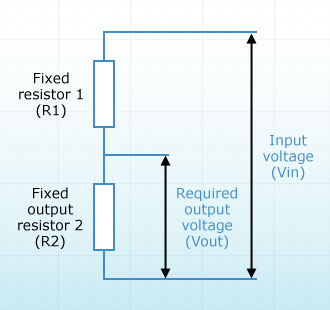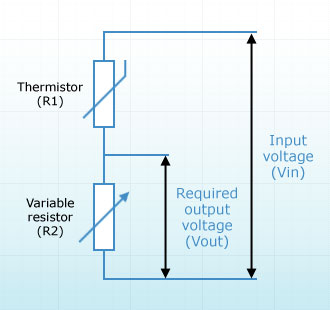You are here: Info Library > Electricity > The Potential Divider
Info Library
Options

# Electricity > The Potential Divider

Potential divider circuits are made so that a particular part of a circuit receives a particular current. They usually consist of two or more resistors that are arranged in series. These resistors can be standard fixed-current types, potentiometers, thermistors, LDRs or a combination of different types.

The diagram below (fig 1) shows a simple two-resistor potential divider circuit showing how resistor two enables the required voltage to be created.Fig 1:
Fixed resistors

The diagram below (fig 2) shows how the same basic circuit can be made to contain a thermistor and a variable resistor. The principles are the same whichever types of resistor you use.Fig 2:
Thermsitor and variable resistor

Potential divider circuits are commonly used in transistor switching circuits where the reduction in voltage across the transistor allows it to 'switch' another part of the circuit. This is useful in light sensor type devices where a light comes on when no light can get to the LDR.